# Civil Engineering - Theory of Structures

### Exercise :: Theory of Structures - Section 1

16.

A load of 1960 N is raised at the end of a steel wire. The minimum diameter of the wire so that stress in the wire does not exceed 100 N/mm2 is :

 A. 4.0 mm B. 4.5 mm C. 5.0 mm D. 5.5 mm E. 6.0 mm

Explanation:

No answer description available for this question. Let us discuss.

17.

The force in BF of the truss shown in given figure, is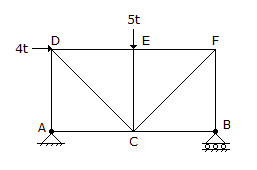A. 4t tension B. 4t compression C. 4.5t tension D. 4.5t compression E. zero.

Explanation:

No answer description available for this question. Let us discuss.

18.

The radius of gyration of a section of area A and least moment of inertia I about the centroidal axis, is

 A.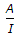B.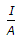C.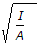D.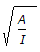Explanation:

No answer description available for this question. Let us discuss.

19.

A simply supported beam carries varying load from zero at one end and w at the other end. If the length of the beam is a, the maximum bending moment will be

 A.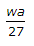B.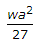C.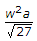D.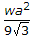Explanation:

No answer description available for this question. Let us discuss.

20.

In plastic analysis, the shape factor for rectangular section, is

 A. 1.4 B. 1.5 C. 1.6 D. 1.7